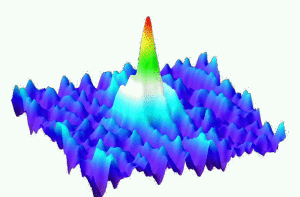In quantum mechanics, the spin-statistics theorem states that sub-atomic particles are either bosons or fermions. Photons are bosons, while electrons are fermions. Bosons have integer spin, and the wave function of a system of two bosons is symmetric. Fermions have half integer spin, and the wave function of a system of two fermions is antisymmetric. Bosons can occupy the same quantum state, and obey to the Bose-Einstein statistics. Fermions cannot occupy the same quantum state (Pauli exclusion principle), and obey to the Fermi-Dirac statistics. These statistics can be retained using some elementary combinatorics, in the spirit of the famous Maxwell-Boltzmann statistics.

Maxwell-Boltzmann statistics. We have ${m}$ energy levels ${E_1,\ldots,E_m}$ and ${n}$ distinguishable particles. If ${n_i}$ is the number of ${E_i}$ particles then ${n=n_1+\cdots+n_m}$ and the average energy of configuration ${(n_1,\ldots,n_m)}$ is ${E=n_1E_1+\cdots+n_mE_m}$. There are

$\binom{n}{n_1,\ldots,n_m}=n!\prod_{i=1}^m\frac{1}{n_i!}$

ways to obtain the configuration ${(n_1,\ldots,n_m)}$ (multinomial coefficient: throw ${n}$ distinguishable balls into ${m}$ boxes). In order to get an additive measurement of the degree of freedom, we may take the logarithm. The additive degree of freedom per particle is then ${\frac{1}{n}\log\binom{n}{n_1,\ldots,n_m}}$. Now, if ${n\rightarrow\infty}$ with ${n_i/n\rightarrow p_i}$ for every ${1\leq i\leq n}$ then ${p_1+\cdots+p_m=1}$, and thanks to the Stirling formula, the asymptotic degree of freedom per particle is given by

$-\sum_{i=1}^mp_i\log(p_i).$

This quantity is known as the Boltzmann entropy. Maximizing this entropy subject to the fixed average energy constraint ${E=\sum_{i=1}^mp_iE_i}$ gives

$p_i=Z^{-1}e^{-\beta E_i}$

where the Lagrange multiplier ${\beta>0}$ can be interpreted to be proportional to an inverse temperature, and were ${Z=\sum_{i=1}^me^{-\beta E_i}}$ is a normalizing factor.

As pointed out by Gibbs, the reasoning above is faulty. Namely, if we consider a system formed by ${n+n’}$ particles, then the logarithmic degree of freedom is actually not additive, violating the second principle of thermodynamics. A way to solve this paradox is to assume that the particles are undistinguishable. This makes the combinatorics trivial since for every configuration ${(n_1,\ldots,n_m)}$ there is a unique way to throw our undistinguishable particles into the ${m}$ boxes with ${n_i}$ particles in each box for every ${1\leq i\leq m}$. To escape from this triviality, we assume that each energy level ${E_i}$ has ${s_i}$ states, in other words that box ${i}$ has ${s_i}$ sub-boxes. The number of ways to obtain configuration ${(n_1,\ldots,n_m)}$ is then

$\prod_{i=1}^m\frac{(n_i+s_i-1)!}{n_i!(s_i-1)!}.$

If ${s_1=\cdots=s_m=1}$ then this number is ${1}$. If ${n_i\ll s_i}$ then thanks to the Stirling formula, the number of ways to obtain configuration ${(n_1,\ldots,n_m)}$ is

$\approx\prod_{i=1}^m\frac{s_i^{n_i}}{n_i!}.$

The variational reasoning used for the distinguishable case leads then to another distribution for the energy levels, known as the Maxwell-Boltzmann statistics:

$p_i\approx Z^{-1}s_ie^{-\beta E_i}.$

When ${s_1=\cdots=s_m}$ we recover the same distribution.

Bose-Einstein statistics. We follow the reasoning used for the Maxwell-Boltzmann statistics, without the assumption ${n_i\ll s_i}$. This leads to

$p_i\approx Z^{-1}\frac{s_i}{e^{\beta E_i-\alpha}-1}.$

Fermi-Dirac statistics. Suppose this time that at most one particle can occupy a state (i.e. a sub-box). The number of ways to obtain configuration ${(n_1,\ldots,n_m)}$ is this time

$\prod_{i=1}^m\frac{s_i!}{n_i!(s_i-n_i)!},$

$p_i\approx Z^{-1}\frac{s_i}{e^{\beta E_i-\alpha}+1}.$